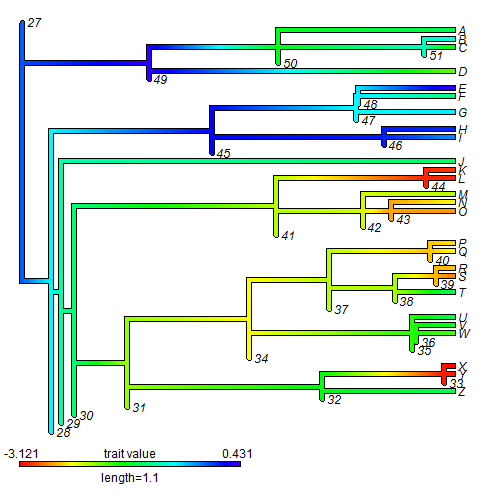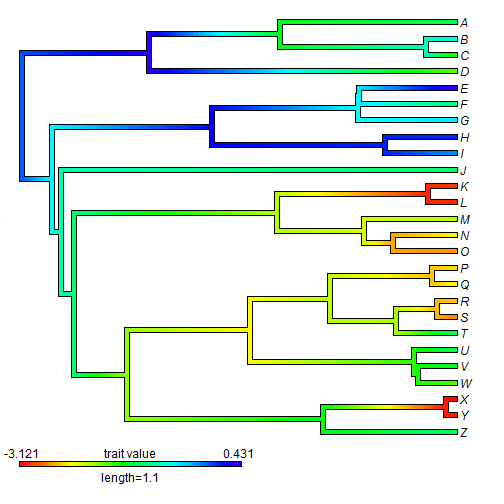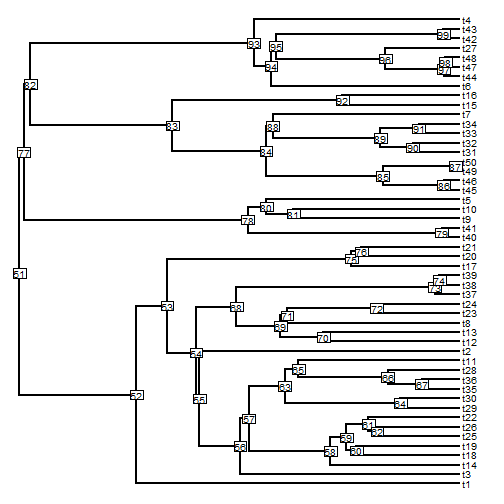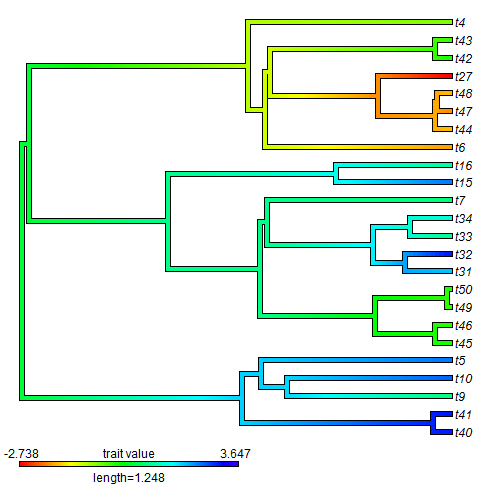## Sunday, August 17, 2014

### drop.tip method for object of class "contMap"; and code to specify internal node values using contMap

A phytools user recently asked me if it is possible to specify the values at some or all internal nodes using the phytools function contMap.

Actually, upon further discussion, what it turns out that she really wanted to do is estimate ancestral states for a larger tree, and then supply those states to contMap.

In either case, it turns out, the easiest way to do this is by first writing a drop.tip method for an object of class "contMap". (I'm using method in the general, not S3 R sense). Then what we're going to do is attach our internal node values as leaves of zero length to internal nodes and run drop.tip.contMap to prune them.

First, this is our drop.tip.conMap function.

drop.tip.contMap<-function(x,tip){
x$tree<-drop.tip.simmap(x$tree,tip)
x
}


Now let's simulate some data & plot the simulated (rather than estimated) states at internal nodes.

library(phytools)
tree<-pbtree(n=26,tip.label=LETTERS[26:1])
x<-fastBM(tree,internal=TRUE)


Finally, let's see how we can use drop.tip.contMap to set values at internal nodes:

## all the nodes in our tree
nodes<-1:tree$Nnode+Ntip(tree) tt<-tree ## go through all nodes & attach a zero-length leaf ## to each node for(i in 1:length(nodes)){ M<-matchNodes(tt,tree) ii<-M[which(M[,2]==nodes[i]),1] tt<-bind.tip(tt,nodes[i],edge.length=0,where=ii) } ## here is our tree with zero-length edges ## normally we would turn off plotting obj<-contMap(tt,x)## now drop these tips obj<-drop.tip.contMap(obj,as.character(nodes)) plot(obj)This can, of course, be modified to set only some internal nodes. Let's also try it with the practical case in which we have a nested subtree that we want to use for contMap, but we would like to use ancestral state estimates obtained for the full tree. This is even easier, because now we just use contMap on the full tree and then drop the tips not in our subtree of interest: set.seed(7) tree<-pbtree(n=50) plotTree(tree,node.numbers=TRUE,fsize=0.8)x<-fastBM(tree) obj<-contMap(tree,x,plot=FALSE) obj<-drop.tip.contMap(obj, setdiff(tree$tip.label,extract.clade(tree,77)\$tip.label))
plot(obj)That's all there is to it. I will add drop.tip.contMap to future versions of phytools.

1.Thank you for this! drop.tip.contMap was exactly what I was hoping for!

I have one more question: When I plot the subclade, the colors still scale to the extremes of the larger clade (ex. 1-20), even if the subclade only spans a portion of that (ex. 1-5). Is it possible to rescale the colors to span only the trait range plotted?

Thank you!

1.Hi Talia.

Yes - this is kind of hard to avoid due to the structure of the "contMap" object in memory. Anything's possible, of course, so I will try to post some code to work around this issue; however in the meantime one option is to estimate the ancestral states for the full tree and then supply them to internal nodes using the trick given in part one of this post. That way the "contMap" range will only span the observed and reconstructed values for the character.

- Liam

2.Yes, that makes sense; I'll give it a shot. Thanks!

1.OK. Let me know if you run into trouble. - Liam

Note: due to the very large amount of spam, all comments are now automatically submitted for moderation.